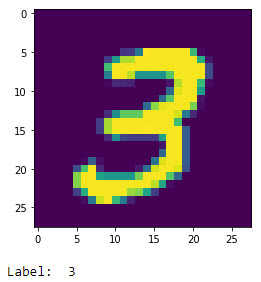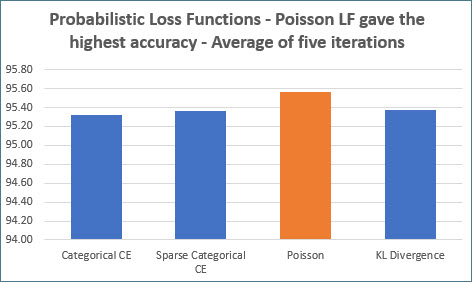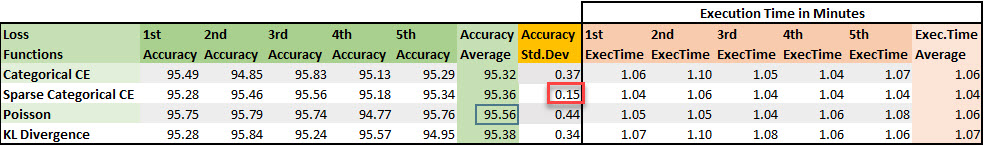# Neural Networks - Types of Classification Loss FunctionsPhoto by Paweł Czerwiński on Unsplash

There are different loss functions available in Keras to predict the output labels.

The loss functions can be regression loss functions for ‘Regression’ type problems and probabilistic loss functions for ‘Classification’ type problems.

This post will take each ‘probabilistic’ loss function and apply it to a classification problem and see how it behaves along with the performance - accuracy and execution time.

### Dataset

Like the previous post here, we will use the MNIST handwritten digits dataset for this classification problem.

MNIST database is a set of images of handwritten digits, and the goal of the deep learning model is to predict the number written correctly. It is a multi-classification problem with digits 0 to 9.

The dataset can be downloaded from here. We will use Keras and the Tensorflow backend for this problem.

• Import the necessary libraries
import matplotlib.pyplot as plt
from sklearn.model_selection import train_test_split
from keras.datasets import mnist
from keras.models import Sequential
from keras.utils.np_utils import to_categorical
from keras.layers import Activation, Dense, BatchNormalization
from keras import optimizers
import numpy as np

• Load the dataset from mnist
(X_train, y_train), (X_test, y_test) = mnist.load_data()

# 11493376/11490434 [==============================] - 7s 1us/step

• Show any number from the dataset to see if it’s working
plt.imshow(X_train)
plt.show()
print('Label: ', y_train)• Let’s reshape the data to convert 28 by 28 pixel data to 1 row by 784 pixels
# reshaping X data: (n, 28, 28) => (n, 784)
X_train = X_train.reshape((X_train.shape, -1))
X_test = X_test.reshape((X_test.shape, -1))

• Let’s split the data to train and test and convert the data to a categorical data
# use only 33% of training data to expedite the training process
X_train, _ , y_train, _ = train_test_split(X_train, y_train, test_size = 0.67,
random_state = 101)

# converting y data into categorical (one-hot encoding)
y_train = to_categorical(y_train)
y_test = to_categorical(y_test)


### Defining a model

Let’s us go ahead and define a model that has

• elu as the first activation function
• softmax as the last function before output (since this is a multi-classification problem)
• 784 as the number of inputs ( 28 by 28 pixels )
• he_normal as the initializer
• 5 layers
• 50 neurons in the first four layers
• 10 neurons in the last layer
• nadam as the optimizer
• accuracy as the metrics
Types of loss functions

• We will use all the following loss functions and check the performance and accuracy it yeilds
• Binary Cross Entropy
• Categorical Cross Entropy
• Sparse Categorical Cross Entropy
• Poisson
• KL Divergence

With the above parameters, let’s define a sequential model.

def mlp_model(activation_first, activation_last, input_shape, initializer,
neurons_layers_first, neurons_layers_last, loss, metrics,
optimizer):
model = Sequential()
model.add(Dense(neurons_layers_first, input_shape = (input_shape , ),
kernel_initializer=initializer))

model.compile(optimizer = optimizer, loss = loss, metrics = metrics)
return model


### Probablistic Loss Functions

#### Binary Cross Entropy

Binary Cross Entropy is usually applied to classification problems when there are just two labels to be predicted.

E.g., we have a set of Cat and Dog pictures, and we need to predict the label as ‘Cat’ or ‘Dog.’ The problem we are analyzing is a binary classification problem as we have just two labels to predict.

The problem that we have in hand is a ‘multi-classification’ problem with the predicted labels ranging from 0 to 9 - a total of ten labels. So, this binary cross-entropy loss function is not the right loss function to be used here.

#### Categorical Cross Entropy

Categorical cross-entropy is used for a ‘multi-classification’ problem.

nadam = optimizers.Nadam(lr = 0.001)
model = mlp_model('sigmoid','softmax', 784 , 'he_normal', 50,10,

%%time
model.fit(X_train, y_train, batch_size = 256, validation_split = 0.3,
epochs = 100, verbose = 0)

# CPU times: user 1min 3s, sys: 3.09 s, total: 1min 6s
# Wall time: 44.6 s
# <tensorflow.python.keras.callbacks.History at 0x7f77578f56d8>

results = model.evaluate(X_test, y_test)
print('Test accuracy: ', results*100)

# 313/313 [==============================] - 0s 2ms/step
#         - loss: 0.3675 - accuracy: 0.9209
# Test accuracy:  92.08999872207642


Using the loss function categorical_crossentropy, we get an accuracy of 92.08

#### Sparse Categorical Cross Entropy

Note

Sparse Categorical Cross Entropy will only work when the target is of one dimension.

In this multi-classification problem, we have one-hot encoded the target variable, and hence Sparse Categorical Cross Entropy will not work.

The following code is run without converting the target variable to one-hot encoding, thereby maintaining the one dimension shape. i.e. retaining the labels as 0,1,2,3,4,5,6,7,8,9 only.

# note that above, we converted y_train to one-hot encoding
# here, we are converting to a single dimension array
y_train = np.argmax(y_train, axis=1)
y_test = np.argmax(y_test, axis=1)

model = mlp_model('sigmoid','softmax', 784 , 'he_normal', 50,10,

%%time
model.fit(X_train, y_train, batch_size = 256, validation_split = 0.3,
epochs = 100, verbose = 0)

# CPU times: user 1min, sys: 2.81 s, total: 1min 3s
# Wall time: 41.7 s
# <tensorflow.python.keras.callbacks.History at 0x7f7751ded5f8>

results = model.evaluate(X_test, y_test)
print('Test accuracy: ', results*100)

# 313/313 [==============================] - 0s 1ms/step
#             - loss: 0.1853 - accuracy: 0.9533
# Test accuracy:  95.32999992370605


Using sparse_categorical_crossentropy, we get an accuracy of 95.33.

#### Poisson

The Poisson loss function gave the highest accuracy so far. More info on Poisson regression here

model = mlp_model('sigmoid','softmax', 784 , 'he_normal', 50,10,

%%time
model.fit(X_train, y_train, batch_size = 256, validation_split = 0.3,
epochs = 100, verbose = 0)

# CPU times: user 1min 2s, sys: 2.86 s, total: 1min 5s
# Wall time: 43.3 s
# <tensorflow.python.keras.callbacks.History at 0x7f774d5295f8>7444b00>

results = model.evaluate(X_test, y_test)
print('Test accuracy: ', results*100)

# 313/313 [==============================] - 0s 1ms/step
# - loss: 0.1200 - accuracy: 0.9575
# Test accuracy:  95.74999809265137


#### KL Divergence

KL divergence loss function applies the Kullback-Leibler divergence algorithm. More info on KL Divergence here

model = mlp_model('sigmoid','softmax', 784 , 'he_normal', 50,10,

%%time
model.fit(X_train, y_train, batch_size = 256, validation_split = 0.3,
epochs = 100, verbose = 0)

# CPU times: user 1min 3s, sys: 3.84 s, total: 1min 7s
# Wall time: 44.7 s
# <tensorflow.python.keras.callbacks.History at 0x7f775216b668>

results = model.evaluate(X_test, y_test)
print('Test accuracy: ', results*100)

# 313/313 [==============================] - 0s 1ms/step
#         - loss: 0.2034 - accuracy: 0.9528
# Test accuracy:  95.27999758720398


We get an accuracy of 95.27 with KL Divergence.

#### Conclusion

Out of all the five loss functions we have seen, we have applied the four-loss functions and have seen how it fared for accuracy.

Here are the lessons learned:

• Binary cross-entropy is to be used for binary classification
• Sparse categorical cross-entropy loss function will work if the output variable is of one dimension
• Do not be shy of using different loss functions to be used if you are tuning the model.

Here are the results of the performance - accuracy and time metrics of different loss functions

• Poisson gave the best accuracy
• Sparse Categorical Cross Entropy gives more reliable accuracy with little standard deviation